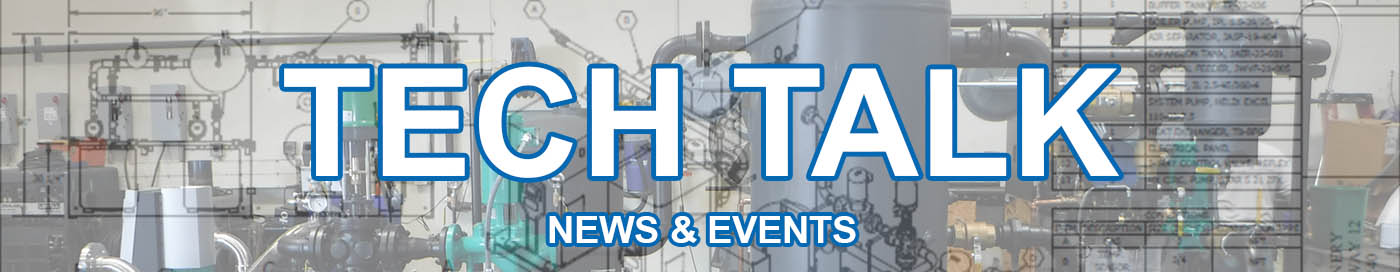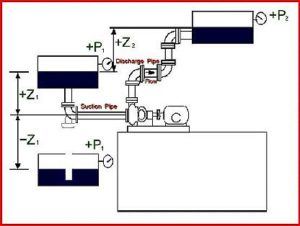NPSH – R.F. MacDonald Co.### How to Calculate TDH & NPSHCALCULATE TOTAL DYNAMIC HEAD (TDH) AND NET POSITIVE SUCTION HEAD (NPSH) The two most critical values that must be calculated for a pump system are Total Dynamic Head (TDH) and Net Positive Suction Head (NPSH). A simple guide to these calculations follows. All symbols noted in the calculations are referenced in the sketch shown above...